# Impact of Fiber Nonlinearity

With the use of dispersion compensation, dispersion ceases to be a limiting factor for lightwave systems. Indeed, the performance of modern long-haul systems is typically limited by the nonlinear effects. In this tutorial, we focus on the techniques used to manage the nonlinear effects.

The use of dispersion management in combination with optical amplifiers can extend the length of a WDM system to several thousand kilometers. If the optical signal is regenerated electronically every 300 km or so, such a system works well as the nonlinear effects do not accumulate over long lengths. In contrast, if the signal is maintained in the optical domain by cascading many amplifiers, the nonlinear effects such as self-phase modulation (SPM) and cross-phase modulation (XPM) ultimately limit the system performance. For this reason, the impact of nonlinear effects on the performance of dispersion-managed systems has been investigated extensively. In this section we study how the nonlinear effects influence a dispersion-managed system and how their impact can be minimized with a suitable choice of system parameters.

#### 1. System Design Issues

In the absence of nonlinear effects, the use of dispersion management ensures that each pulse is confined to its bit slot when the optical signal arrives at the receiver, even if pulses have spread over multiple slots during their transmission. Moreover, it is immaterial whether dispersion is compensated at the transmitter end, receiver end, or periodically within the fiber link. Any dispersion map can be used as long as the accumulated group-velocity dispersion (GVD) vanishes at the end of a link of length L:The performance of such a systems is only limited by the SNR degradation induced by amplifier noise. Since SNR can be improved by increasing input optical power, link length can be made arbitrarily long in principle.

However, the nonlinear effects are not negligible for long-haul WDM systems when channel powers exceed a few milliwatts, and the transmission distance is often limited by the nonlinear effects. Moreover, as seen in the periodically amplified lightwave systems tutorial, an optimum power level exists at which the system performs best. Dispersion management is essential for long-haul systems to ensure that the system is not limited by GVD-induced pulse broadening. However, different dispersion maps can lead to different Q factors at the receiver end even when da = 0 for all of them. This is so because the dispersive and nonlinear effects do not act on the signal independently. More specifically, degradation induced by the nonlinear effects depends on the local value of da(z) at any distance z within the fiber link.

The major nonlinear phenomenon affecting the performance of a single isolated channel is the SPM. The propagation of an optical bit stream inside a dispersion-managed system is governed by the NLS equation that can be written as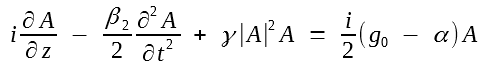where we have ignored the noise term to simplify the following discussion. In a dispersion-managed system, the three fiber parameters (β2, γ, and α) are functions of z because of their different values in two or more fiber sections used to form the dispersion map. The gain parameter g0 is also a function of z because of loss management. Its functional form depends on whether a lumped or a distributed amplification scheme is employed.

The above equation is solved numerically to analyze the performance of dispersion-managed systems. As was done in the compensation of fiber losses tutorial, it is useful to eliminate the gain and loss terms in this equation with the transformationand write it in terms of U(z, t) aswhere P0 is the input peak power and p(z) governs variations in the peak power of the signal along the fiber link throughIf losses are compensated in a periodic fashion, p(zm) = 1, where zm = mLA is the location of the mth amplifier and LA is the amplifier spacing. In the case of lumped amplifiers, g0 = 0 within the fiber link, andThe effective nonlinear parameter γe ≡ γp(z) is also z-dependent because of changes in the signal power induced by fiber losses and optical amplifiers. In particular, when lumped amplifiers are sued, the nonlinear effects are strongest just after signal amplification and become negligible in the tail end of each fiber section between two amplifiers.

There are two major design issues for any dispersion-managed system: What is the optimum dispersion map and which modulation format provides the best performance? Both of these issues have been studied by solving the NLS equation above numerically. Although attention was initially focused on the NRZ format, the performance of RZ and NRZ formats was compared starting in 1996, both numerically and experimentally, under realistic operating conditions. As an example, the figure below shows the numerical results for the (a) NRZ and (b) RZ format by plotting the maximum transmission distance L at which eye opening is reduced by 1 dB at the receiver of a 40-Gb/s system as average launched power is increased. The periodic dispersion map consisted of 50 km of standard fiber with D = 16 ps/(km-nm), α = 0.2 dB/km, and γ = 1.31 W-1/km, followed by 10 km of dispersion-compensating fiber (DCF) with D = -80 ps/(km-nm), α = 0.5 dB/km, and γ = 5.24 W-1/km. Optical amplifiers with 6-dB noise figure were placed 60 km apart and compensated total fiber losses within each map period. The duty cycle was 50% in the case of the RZ format.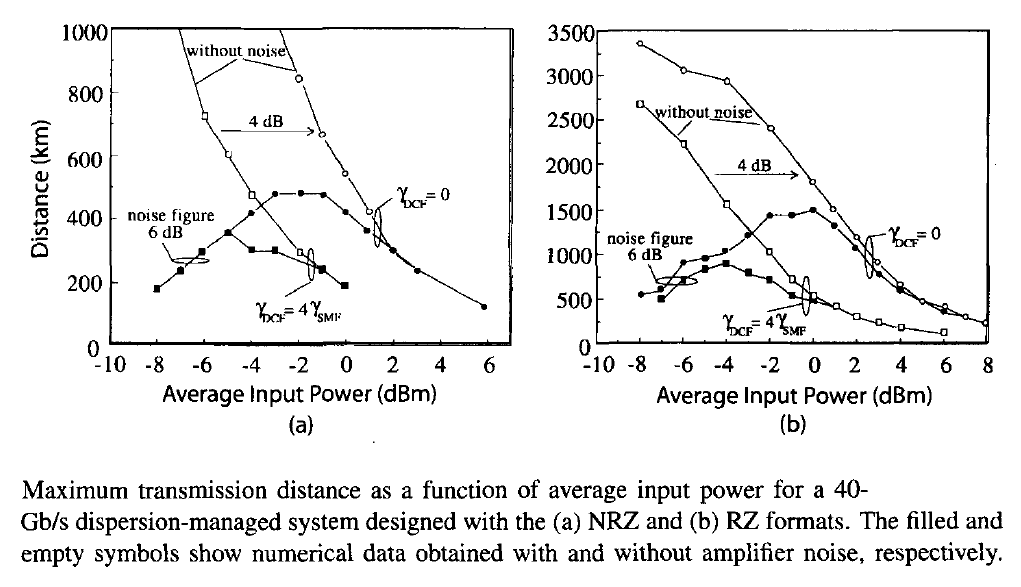As evident from the figure above, distance can be continuously increased in the absence of amplifier noise by decreasing the launched power (open squares). However, when noise is included, an optimum power level exists for which the link length is maximum. This distance is less than 400 km when the NRZ format is employed but becomes larger by about a factor of 3 when the RZ format is implemented with a 50% duty cycle. The reason behind this improvement can be understood by noting that the dispersion length is relatively small (<5 km) for RZ pules propagating inside a standard fiber. As a result, RZ format pulses spread quickly and their peak power is reduced considerably compared with the NRZ case. This reduction in the peak power lowers the impact of SPM.

The figure above also shows how the buildup of nonlinear effects within DCFs affects the system performance. In the case of RZ format, maximum distance is below 900 km at an input power level of -4 dBm because of the DCF-induced nonlinear degradation (filled squares). Not only DCFs have a larger nonlinear parameter because of their smaller core size, pulses are also compressed inside them to their original width, resulting in much higher peak powers. If the nonlinear effects can be suppressed within DCF, maximum distance can be increased close to 1,500 km by launching higher powers. This improvement can be realized in practice by using an alternate dispersion-compensating device requiring shorter lengths (such as a two-mode DCF or a fiber grating). In the case of NRZ format, the link length is limited to below 500 km even when nonlinear effects are negligible within DCFs.

As seen from the figure above, the nonlinear effects play an important role in dispersion-managed systems whenever a DCF is used because its smaller core size enhances optical intensities (manifested through a larger value of the γ parameter). Placement of the amplifier after the DCF helps since the signal is then weak enough that the nonlinear effects are less important in spite of a small core area of DCFs. The optimization of system performance using different dispersion maps has been the subject of intense study. In a 1994 experiment, a 1,000-km-long fiber loop containing 31 fiber amplifiers was used to study three different dispersion maps. The maximum transmission distance of 12,000 km at a bit rate of 5 Gb/s was realized for the case in which short section s of normal-GVD fibers were used to compensate for the anomalous GVD of long sections. In a 1995 experiment, the transmission distance of a 80-Gb/s signal, obtained by multiplexing eight 10-Gb/s channels with 0.8-nm channel spacing, was found to be limited to 1,171 km because of the onset of various nonlinear effects.

The choice between the RZ and NRZ formats is not always so obvious as it depends on many other design parameters. As early as 1995, an experiment as well as numerical simulations indicated that a 10-Gb/s signal can be transmitted over 2,245 km using the NRZ format with 90-km amplifier spacing, provided the link dispersion is not fully compensated. A similar conclusion was reached in a 1999 experiment in which RZ and NRZ formats were compared for a 10-Gb/s system. The following figure shows the recirculating loop used in this experiment. Because of cost considerations, most laboratory experiments employ a fiber loop in which the optical signal is forced to recirculate many times to simulate a long-haul lightwave system. Two optical switches determine how long a pseudorandom bit stream circulates inside the loop before it reaches the receiver. The loop length and the number of round trips set the total transmission distance. The loop shown in the figure below contains two 102-km sections of standard fiber and two 20-km DCFs. A filter with a 1-nm bandwidth reduces the buildup of broadband ASE noise. The 10-Gb/s signal could be transmitted over 2,040 km with both the RZ and NRZ formats when launched power was properly optimized. However, it was necessary to add a 38-km section of standard fiber in front of the receiver in the NRZ case so that dispersion was not fully compensated.Perfect compensation of GVD in each map period is not generally the best solution in the presence of nonlinear effects. A numerical approach is generally used to optimize the design of dispersion-managed lightwave systems. In general, local GVD should be kept relatively large, while minimizing the average dispersion for all channels. In a 1998 experiment, a 40-Gb/s signal was transmitted over 2,000 km of standard fiber using a novel dispersion amp. The distance could be increased to 16,500 km at a lower bit rate of 10 Gb/s by placing an optical amplifier right after the DCF within the recirculating fiber loop. Since the nonlinear effects were not negligible, it is believed that soliton properties played an important role in this experiment.

A systematic study based on the NLS equation shows that although the NRZ format can be used at 10 Gb/s, the RZ format is superior in most practical situations for lightwave systems operating at bit rates of 40 Gb/s or higher. Even at 10 Gb/s, the RZ format can be used to design systems that are capable of transmitting data over a distance of up to 10,000 km over standard fibers. Such a performance is not realizable with the NRZ format. For this reason, the focus of this tutorial is high-speed systems designed with the RZ format.

#### 2. Semianalytic Approach

Although the NLS equation must be solved numerically for a realistic modeling of lightwave systems, considerable insight can be gained by adopting a semianalytic approach in which the dispersive and nonlinear effects are considered for a single optical pulse representing an isolated 1 bit. In this case, the NLS equation can be reduced to solving a set of two ordinary differential equations using a variational approach. The moment method can also be employed for this purpose. Both methods assume that each optical pulse maintains its shape even though its amplitude, width, and chirp may change during propagation. As seen in the dispersion-induced pulse broadening tutorial, a chirped Gaussian pulse maintains its functional form in the linear case (γ = 0). If the nonlinear effects are relatively weak in each fiber section compared with the dispersion effects, the pulse is likely to retain its Gaussian shape approximately even when nonlinear effects are included.

At a distance z inside the fiber, the envelop of a chirped Gaussian pulse has the formwhere a is the amplitude, T is the width, C is the chirp, and φ is the phase. All four parameters vary with z. The variational or the moment method can be used to obtain four ordinary differential equations governing the evolution of these four parameters along the fiber link. The phase equation can be ignored as it is not coupled to the the other three equations. The amplitude equation can be integrated to find that the product a2T does not vary with z and is related to the input pulse energy E0 as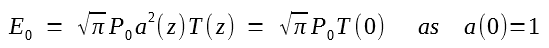Thus, we only need to solve the following two coupled equations:Details of loss and dispersion managements appear in these equations through the z dependence of three parameters β2, γ, and p. The two equations above require values of three pulse parameters at the input end, namely the width T0, chirp C0, and energy E0, before they can be solved. The pulse energy E0 is related to the average power launched into the fiber link through the relation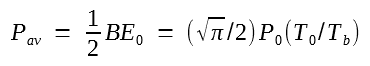where Tb is the duration of bit slot at the bit rate B.

Consider first the linear case by setting γ(z) = 0. In this case, E0 plays no role because pulse-propagation details are independent of the initial pulse energy. The two equations above can be solved analytically in the linear case and have the following general solution: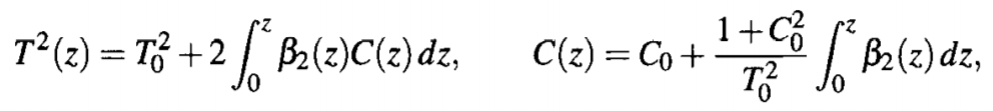where details of the dispersion map are included through β2(z). This solution looks complicated but it is easy to perform integrations for a two-section dispersion map. The values of T and C at the end of the map period z = Lmap are given by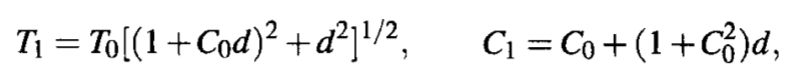where the dimensionless parameter d is defined as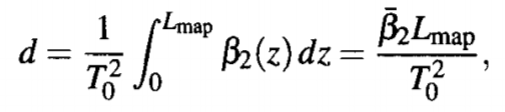and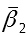is the average value of the dispersion parameter over the map period Lmap.

As evident from the T1 and C0 solution above, when nonlinear effects are negligible, the final pulse parameters depend only on the average dispersion, and not on details of the dispersion map. This is exactly what one would expect when the theory of nonlinear optical effects tutorial is applied to a linear system. If the dispersion map is designed such that= 0, both T and C return to their input values at z = Lmap. In the case of a periodic dispersion map, each pulse would recover its original shape after each map period if d = 0. However, when the average GVD of the dispersion-managed link is not zero, T and C change after each map period, and pulse evolution is not periodic.

To study how the nonlinear effects governed by the γ term affect the pulse parameters, we can solve the equation below numerically.The following figure shows the evolution of pulse width and chirp over the first 60-km span for an isolated pulse in a 40-Gb/s bit stream using the same two-section dispersion map employed for the first figure (50-km standard fiber followed with 10 km of DCF). Solid lines represent 10-mW launched power. Dotted lines show the low-power case for comparison. In the first 50-km section, pulse broadens by a factor of about 15, but it is compressed back in the DCF because of dispersion compensation. Although the nonlinear effects modify both the pulse width and chirp, changes are not large even for a 10-mW launched power. In particular, the width and chirp are almost recovered after the first 60-km span.The situation changes considerably if the pulse is allowed to propagate over many map periods. The following figure shows the pulse width and chirp after each amplifier (spaced 60-km apart) over a distance of 3,000 km (50 map periods). At a relatively low power level of 1 mW, the input values are almost recovered after each map period as dispersion is fully compensated. However, as the launched power is increased beyond 1 mW, the nonlinear effects start to dominate, and the pulse width and chirp begin to deviate considerably from their input values, in spite of dispersion compensation. Even for Pav = 5 mW, pulse width becomes larger than the bit slot after a distance of about 1,000 km, and the situation is worse for Pav = 10 mW. Thus, the optimum power level is close to 1 mW if the objective is to minimize the impact of nonlinear effects. This conclusion is in agreement with the results shown in the first figure above and obtained by solving the NLS equation directly, after including the effect of amplifier noise.

#### 3. Soliton and Pseudo-linear Regimes

As the preceding discussion shows, when the nonlinear term is not negligible, pulse parameters do not return to their input values after each map period even for perfect dispersion compensation (d = 0). Eventually, the buildup of nonlinear distortion affects each pulse within the optical bit stream so much that the system cannot operate beyond a certain distance. As seen in the first figure in this tutorial, this limiting distance can be under 500 km depending on the system design. For this reason, the management of nonlinear effects is an important issue for long-haul lightwave systems. It turns out that the parameters associated with a dispersion map (length and GVD of each section) can be controlled to manage the nonlinearity problem. Two main techniques have evolved, and systems employing them are said to operate in the pseudo-line and soliton regimes.

It was noted in several experiments that a nonlinear system performs best when GVD compensation is only 90% to 95% so that some residual dispersion remains after each map period. In fact, if the input pulse is initially chirped such that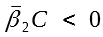, the pulse at the end of the fiber link may even be shorter than the input pulse. This behavior is expected for a linear system. It also persists for weakly nonlinear systems. This observation has led to the adoption of the chirped RZ (CRZ) format for dispersion-managed fiber links.

To understand how the system and fiber parameters affect the evolution of an optical signal inside a fiber link, consider a lightwave system in which dispersion is compensated only at the transmitter and receiver ends. Since fiber parameters are constant over most of the link, it is useful to introduce the dispersion and nonlinear length scales asrespectively. Introducing a normalized time τ as τ = t/T0, the NLS equation can be written in the formwhere s = sign(β2) = ±1, depending on the sign of β2. If we use γ = 2 W-1/km as a typical value, the nonlinear length LNL ~ 100 km at peak-power levels in the range of 2 to 4 mW. In contrast, the dispersion length LD can vary over a wide range (from ~1 to 10,000 km), depending on the bit rate of the system and the type of fibers used to construct it. As a result, one can encounter the following three situations.

If LD ≫  LNL and link length L < LD, the dispersive effects play a minor role, but the nonlinear effects cannot be ignored when L > LNL. This is the situation for systems operating at a bit rate of 2.5 Gb/s or less. For example, LD exceeds 1,000 km at B = 2.5 Gb/s even for standard fibers with β2 = -21 ps2/km and can exceed 10,000 km for dispersion-shifted fibers. Such systems can be designed to operate over long distance by reducing the peak power and increasing the nonlinear length accordingly. The use of a dispersion map is also helpful for this purpose.

If LD and  LNL are comparable and much shorter than the link length, both the dispersive and nonlinear terms are equally important in the NLS equation. This is often the situation for 10-Gb/s systems operating over standard fibers because LD becomes ~100 km when T0 is close to 50 ps. The use of optical solitons is most beneficial in the regime in which LD and  LNL have similar magnitudes. a soliton-based system confines each pulse tightly to its original bit slot by employing the RZ format with a low duty cycle and maintains this confinement through a careful balance of frequency chirps induced by GVD and SPM. Since GVD is used to offset the impact of nonlinear effects, dispersion is never fully compensated in soliton-based systems. It will be seen in the next tutorial that solitons can be formed only in the anomalous-GVD regime. A dispersion map can still be used but the average dispersion of the fiber link should be controlled. Solitons formed under such conditions are known as dispersion-managed solitons and are covered in the next tutorial.

If LD ≪  LNL, we enter a new regime in which dispersive effects dominate locally, and the nonlinear effects can be treated in a perturbative manner.  This situation is encountered in lightwave systems whose individual channels operate at a bit rate of 40 Gb/s or more. The bit slot is only 25 ps at 40 Gb/s. If T0 is < 10 ps and standard fibers are employed, LD is reduced to below 5 km. A lightwave operating under such conditions is said to operate in the quasi-linear or pseudo-linear regime. In such systems, input pulses broaden so rapidly that they spread over several neighboring bits. The extreme broadening reduces their peak power by a large factor. Since the nonlinear term in the NLS equation scales with the peak power, its impact is considerably reduced. Interchannel nonlinear effects are reduced considerably in pseudo-linear systems because of an averaging effect that produces a nearly constant total power in all bit slots. In contrast, overlapping of neighboring pulses enhances the intrachannel nonlinear effects. As nonlinear effects remain important, such systems are called pseudo-linear. Of course, pulses must be compressed back at the receiver end to ensure that they occupy their original time slot before the optical signal arrives at the receiver. This can be accomplished by compensating the accumulated dispersion with a DCF or another dispersion-equalizing filter.